Radial and angular covariances in the study of...

...Spatial Heterogeneity

Please bear with us as links to this topic, which has been in the background for some time, are consolidated on this page. The archived version of the tutorial (PDF), prepared for application to compositional data from highly unequilibrated and fine-grained chondritic interplanetary dust, may be found at...

• "Heterogeneity, and the secret of the sea", arXiv:cond-mat/0403013
P. Fraundorf (UM-StL Physics and Astronomy/CME)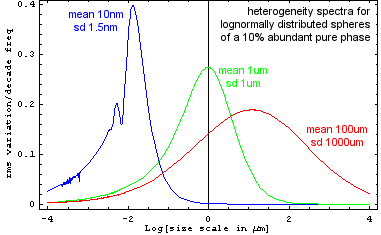If one's (real) random variables are defined on an n-dimensional continuum x, the cross-covariance of variables A[x] and B[x] as a function of n-dimensional vector displacement (or lag) Δx may be defined as σAB[Δx]≡<(A[x+Δx]-μA)(B[x]-μB)>x. Here the population (as distinct from sample) average over x is denoted by angle brackets <>x or the Greek letter μ.

This quantity, called a second-moment correlation measure because it's a generalization of the second-moment statistic variance to variables sampled at known locations x on an n-dimensional manifold, is sometimes put into dimensionless form by normalizing with the population standard deviations of A and B (e.g. σA≡Sqrt[σAA]). This results in a correlation coefficient ρAB[Δx] ≡ σAB[Δx]/(σAσB) that takes on values between plus and minus one. When A is the same as B, the foregoing expressions yield values for autocovariance, a quantity also known in scattering theory as the pair-correlation (or Patterson) function.

The Appendix above shows, perhaps for the first time, that if one defines sample bias coefficient ρ as an average of the autocorrelation-coefficient ρAA[Δx] over all point pairs in a set of M sample points, an unbiased estimate for expected error in the (sample estimate of the population) mean of A is the square root of: sample variance (taken as a population) times (1+(M-1)ρ)/((M-1)(1-ρ)). The derivation is robust in that it does not depend on bell-curve or related assumptions about the distribution of errors.

When ρ is positive and much greater than 1/(M-1), this reduces to the square root of: sample variance (taken as a population) times ρ/(1-ρ). When ρ is negative and much less than -1/(M-1), an upper limit on M asserts itself (M<1-1/ρ) since more than two sample points cannot all be anti-correlated. Finally, when |ρ| is near zero and much less than 1/(M-1) this yields the more familiar expression for standard error, namely the square root of: sample variance (taken as a population) over (M-1). The ρ=0 line on the plot below (with a log-log slope of -˝) is this standard error for an unbiased sample.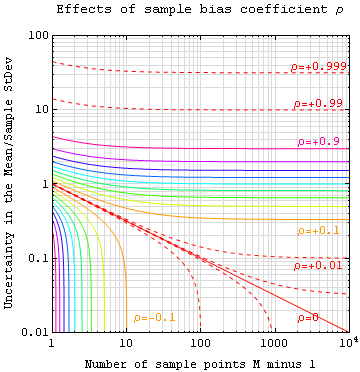Notes: The "Sample StDev" mentioned in the vertical axis label is the square root of sample variance (treated as a population), rather than the ρ=0 sample-based estimate of population standard deviation, which differs from the former by a factor of Sqrt[M/(M-1)]. The unbiased nature of this error-in-the-mean estimate is shown valid for arbitrary samples in Appendix 5 of P. Fraundorf (1980) "Microcharacterization of interplanetary dust collected in the earth's stratosphere" (Ph.D. Dissertation in Physics, Washington University, Saint Louis MO).

Postscript: Replacing M above with N and putting the result through Wikipedia's LaTeX processor, limiting cases for mean square error in terms of sample variance sAA might look like: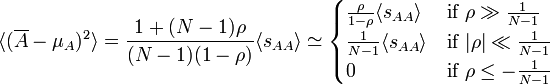Below, the bias coefficient is put to an experimental test using the two dimensional 256×256 dataset on the left. Its Fourier transform is shown in logarithmic complex color to the right of that, followed by a plot of the power spectrum's normalized inverse transform or correlation-coefficient matrix, also in complex color (red = real positive, cyan = real negative). Finally, the graph at right plots analyses for square data patches of varying size whose upper left corner is located at two different points: red=(2,2) and blue=(25,25). The red and blue solid lines traverse mean value measurements for patches of the given size, with error bars reflecting uncertainty in the estimate of population average (the exact value is zero) based on population measurements of the sample bias coefficient. This also means that we can now do this test with any data set that you'd like to try it with. The gray lines represent the much smaller error bars predicted by the ρ=0 standard error.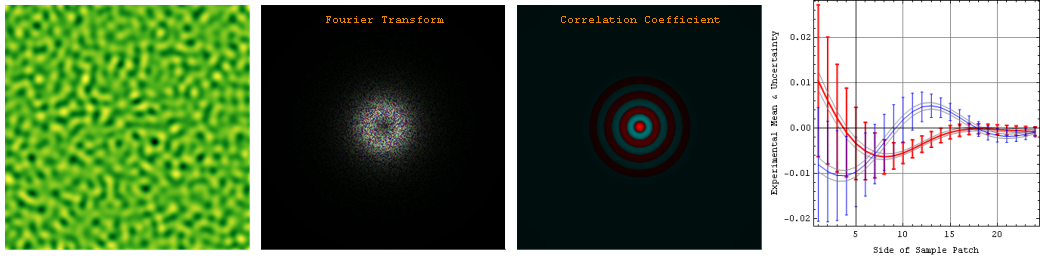The Appendix above also describes an approximate "cube containment model" for the sample bias coefficient of a specimen with grain size d sampled over a region of size D.Below we compare the predictions of that model to some experimental analyses.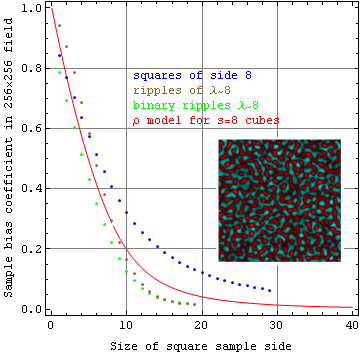The binary ripples specimen shown below is our analog of a rock containing equal abundances of two minerals. It was generated by segmenting a non-binary specimen with mean wavelength of 8 pixels, like the one used above. Not only are the grains not square, but the distribution probabilities are also radically non-Gaussian. Below that, you'll find the size-8 squares specimen, whose intensity distributions are equally non-Gaussian. All of these images in logarithmic complex color, where red specifies +1, cyan -1, chartreuse i, indigo -i, black zero, and white ±∞.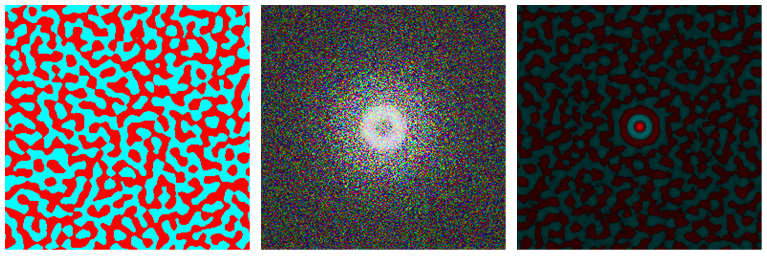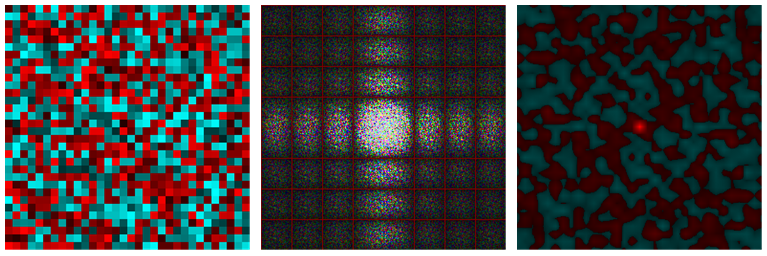Beyond the expected error in one's estimate of the population mean from a limited sample, the distribution of such errors in a specimen with non-zero bias may also be of interest. The bias, in effect, will modify predictions of the unbiased Student T distribution (at least for the case when normally distributed errors is a useful approximation). The figure below illustrates the distribution of errors with respect to a zero mean for 625 square sample regions each 10 pixels on a side, taken from a 256×256 λ=8 ripple specimen like that above. The experimental sample bias coefficient for this sample size was ρ=0.223. The red line shows the student T distribution expected for an unbiased specimen. Deviations from the unbiased prediction are not particularly noticeable in this case, although that's hardly a surprise given that the mean, standard deviation (error in the mean), and normalization are specified going in. The first-order effect of sample bias is thus to modify the size of the error, and not the shape of the error distribution.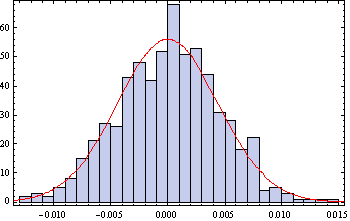...Surface Roughness

Two notes on roughness spectroscopy (with a focus on SPM data) may be found at...

Old notes with some quite detailed equations on the same subject can be found here.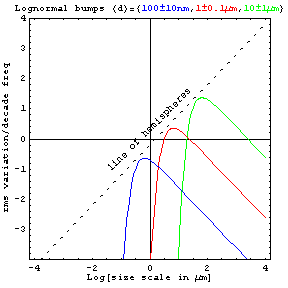Below is are 1x and 4x greyscale maps of topography on a specimen with some interesting roughness structure. Do you notice anything unusual about it, and what do you think it's roughness spectrum looks like?

1x: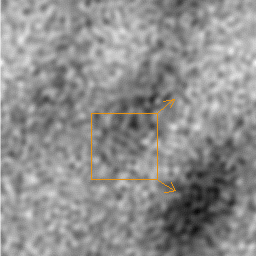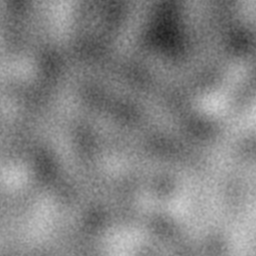:4x

Here's the 1x map rendered in 3d. What can you say about processes that operated to build this topography?...Lattice Fringe Images

Some discussion of angular covariance in analysis of HREM TEM images may be found in our digital interferometry papers, posters, and talks for Microscopy and Microanalysis 2004 August/Savannah GA.

More on fringe correlation maps will be posted here.

A large number of interactive fringe visibility maps may be found here.